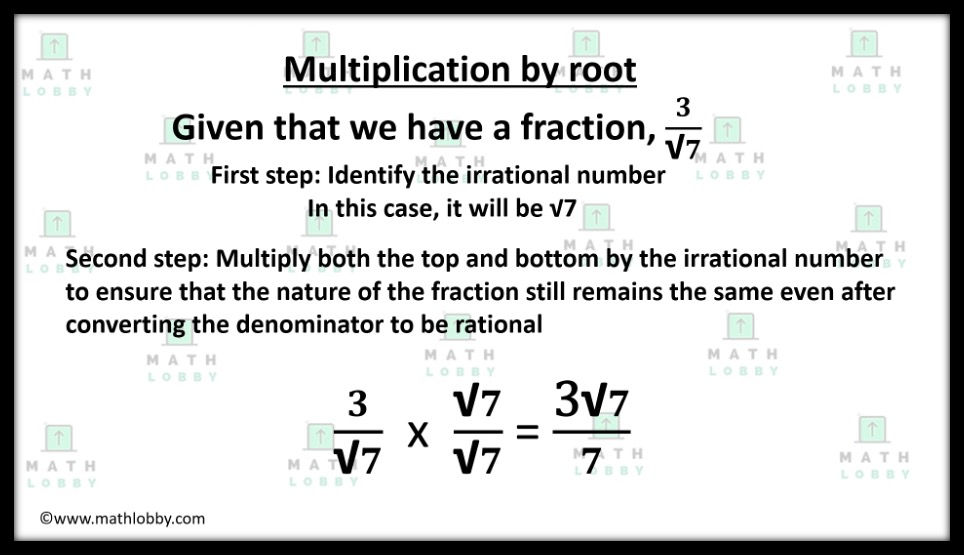top of page
Search

# Surds Part 2- Rationalization

Updated: Jun 22, 2021Dear Secondary Math students, in our previous article, we covered the Basic of surds and different laws on how to manipulate surds. Make sure to click on the link above and go through it before proceeding to Rationalization. As for today, we will be covering Rationalization Of Surds. Without further ado, let's begin!

In this note, you will learn:

1) What is rationalization?

2) The purpose of rationalization

### 1) What is rationalization?

Rationalization is essentially the act of converting the denominator of a fraction from an irrational number to become a rational number. This typically involves surds, which are square or cube roots of a number and can no longer be simplified further.

The way to do this is through the manipulation of the fraction and shifting the irrational denominator to become the numerator.

### 2) The purpose of rationalization

So, why is rationalizing important when we are dealing with questions involving surds? Although there is nothing wrong with having an irrational number to be the denominator of a fraction, but when questions specifically state that they want your answers to be given in the “simplest form”.

Therefore, when the denominator of a fraction contains a surd, it will be deemed as irrational and your answers are hence not in the simplest form as it can be.

This will cause you to lose marks in examinations!

Now, we have two methods of approach to rationalize a denominator of a fraction:

· Multiplication of both top and bottom of the fraction by the root

· Multiplication of both top and bottom of the fraction by the conjugate surd

The first method we will be talking about is the multiplication of both top and bottom of the fraction by the root. What we mean by this is that if we are able to multiply the root by itself, by the Laws of Surds, we will end up with a rational number at the bottom!

However, that is only part of the solution. Although we have now made the denominator rational, we must also remember to not change the nature of the initial answer, hence we will have to multiply the top of the fraction by the same root as well!

Let’s take a look at the example given below:Given that we have a fraction 3/√7, the first step is to identify the irrational number, which in this case is √7 and it is in the denominator.

Secondly, we will then multiply both the top and bottom of the fraction by the irrational number, because manipulation to both the top and bottom of the fraction will ensure that the nature of the fraction remains the same. So, (3/√7) x (√7/√7)

And therefore, we will end up with: (3√7)/7, which is the simplest form of the fraction.

The second method we will be talking about will be the multiplication of both top and bottom of the fraction by the conjugate surd.

This method is typically used when we are dealing with a more complex irrational denominator, and how this works is that we must recall the formula back from the basics of algebra and manipulation, (x + y) (x – y) = x²– y²!

If you are unclear of the basics of algebra and its manipulation, Math Lobby has an article dedicated specifically for it. Click on the link above to check it out!

Regardless of whether the irrational number is in the ‘x’ or ‘y’ term, when we multiply by its conjugate (If you are unclear of the concept on conjugate roots, the end form will always end with a square for both the terms, which will result in the negation of the square root of the surd!

Let’s take a look at the following example:Given that we have a fraction 1/ (5 - 2√3), the first step is similar to the first step in the first method, but instead of just taking the surd, we must take the whole denominator. i.e. 5 - 2√3

Secondly, we will identify the conjugate of the irrational denominator, in this case 5 + 2√3, and multiply both the top and bottom by the conjugate of the irrational denominator.

This is because we want the end form of the irrational denominator to end up being rational by the manipulation of the algebraic formula: (x + y) (x – y) = x²– y². So,

[1/ (5 - 2√3)] x [(5 + 2√3)/ (5 + 2√3)]

And therefore, we will end up with: (5 + 2√3)/13, which is the simplest form of the fraction.

Question Time!

Rationalize the following fractions into its simplest forms

a) 4 / (6 - √3)

b) 5 / √8

c) 3 / (√5 + 7)

d) 6 / √3

e) 9 / (4√2 + 8)

f) 5 / 7√8

g) 1 / (3√11 – 10)

h) 3 / 8√2

And that’s all for today, students! Math Lobby hopes that after this article, you have a clear understanding on the rationalization of irrational denominators, and how useful this skill can be in solving questions involving surds in the denominators of fractions!

If you have any pending questions, please do go on to our Facebook page, Instagram or contact us directly at Math Lobby! We have certified mathematics tutors to aid you in your journey to becoming a better student!

As always: Work hard, stay motivated and we wish all students a successful and enjoyable journey with Math Lobby!

If you want to receive more Secondary Math Tips from us,

Visit and FOLLOW our Instagram page at https://www.instagram.com/mathlobbymotivation/